Chapter 3 Stoichiometry Calculations with Chemical Formulas and

• Slides: 53Chapter 3 Stoichiometry: Calculations with Chemical Formulas and Equations Stoichiometry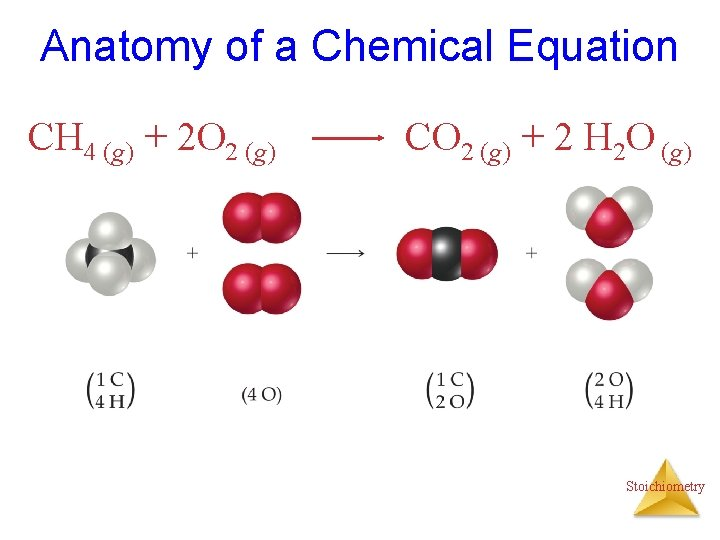Anatomy of a Chemical Equation CH 4 (g) + 2 O 2 (g) CO 2 (g) + 2 H 2 O (g) StoichiometryAnatomy of a Chemical Equation CH 4 (g) + 2 O 2 (g) Reactants appear on the left side of the equation. CO 2 (g) + 2 H 2 O (g) StoichiometryAnatomy of a Chemical Equation CH 4 (g) + 2 O 2 (g) CO 2 (g) + 2 H 2 O (g) Products appear on the right side of the equation. Stoichiometry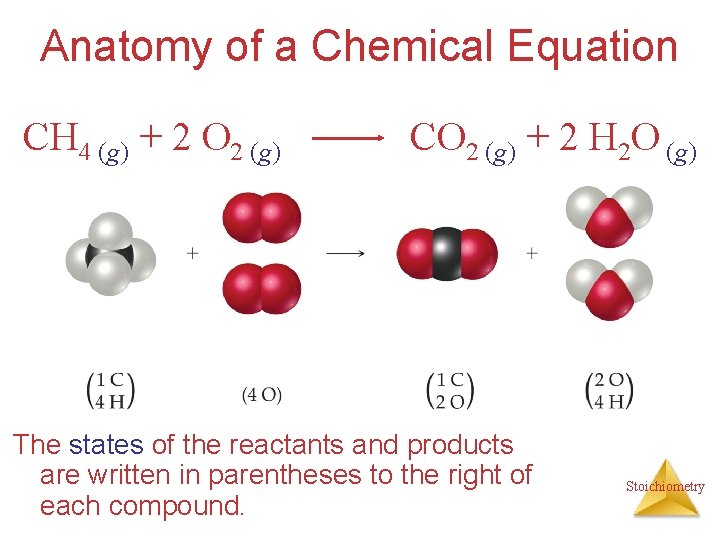Anatomy of a Chemical Equation CH 4 (g) + 2 O 2 (g) CO 2 (g) + 2 H 2 O (g) The states of the reactants and products are written in parentheses to the right of each compound. Stoichiometry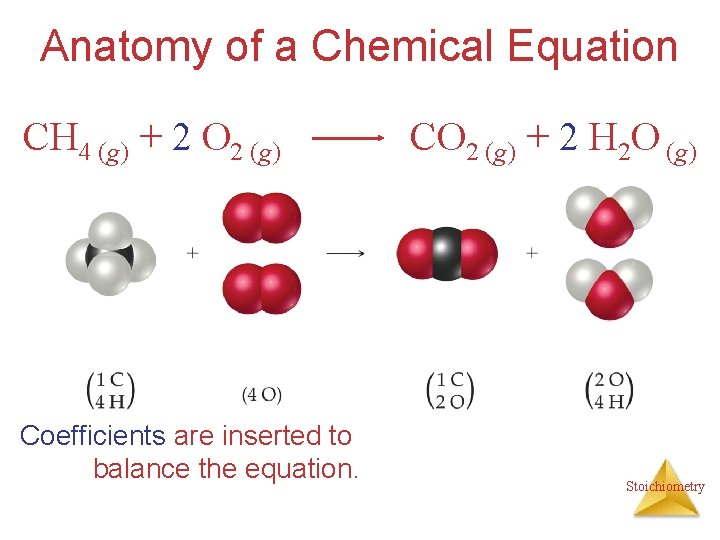Anatomy of a Chemical Equation CH 4 (g) + 2 O 2 (g) Coefficients are inserted to balance the equation. CO 2 (g) + 2 H 2 O (g) Stoichiometry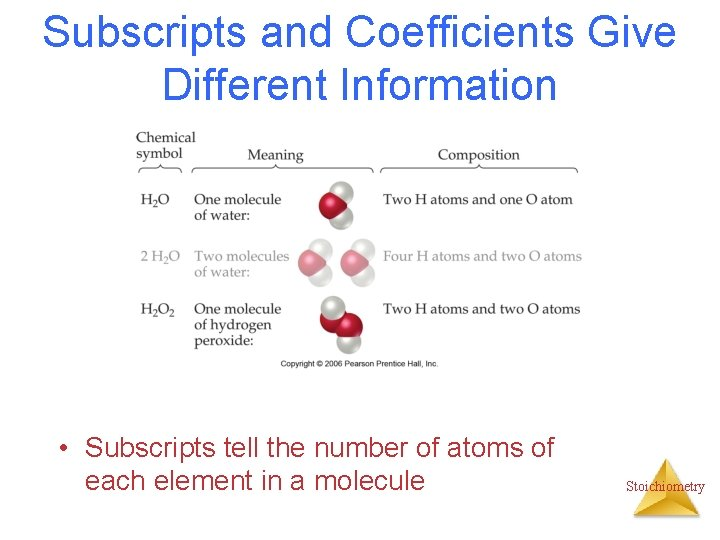Subscripts and Coefficients Give Different Information • Subscripts tell the number of atoms of each element in a molecule StoichiometrySubscripts and Coefficients Give Different Information • Subscripts tell the number of atoms of each element in a molecule • Coefficients tell the number of molecules (compounds). StoichiometryReaction Types Stoichiometry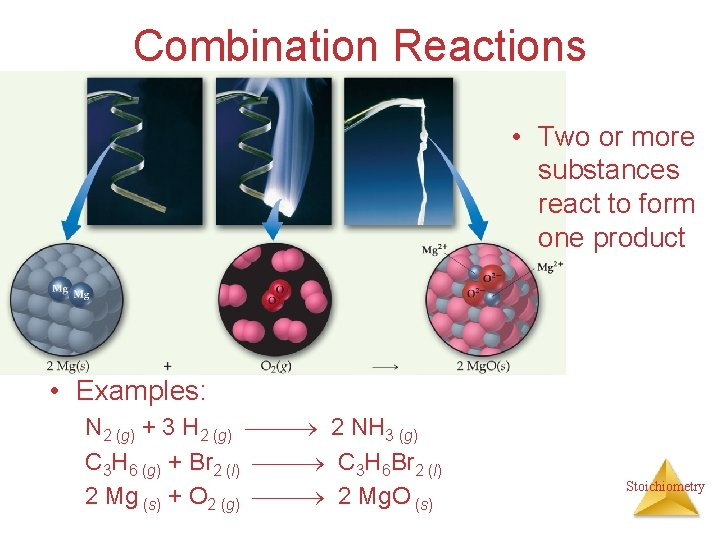Combination Reactions • Two or more substances react to form one product • Examples: N 2 (g) + 3 H 2 (g) 2 NH 3 (g) C 3 H 6 (g) + Br 2 (l) C 3 H 6 Br 2 (l) 2 Mg (s) + O 2 (g) 2 Mg. O (s) Stoichiometry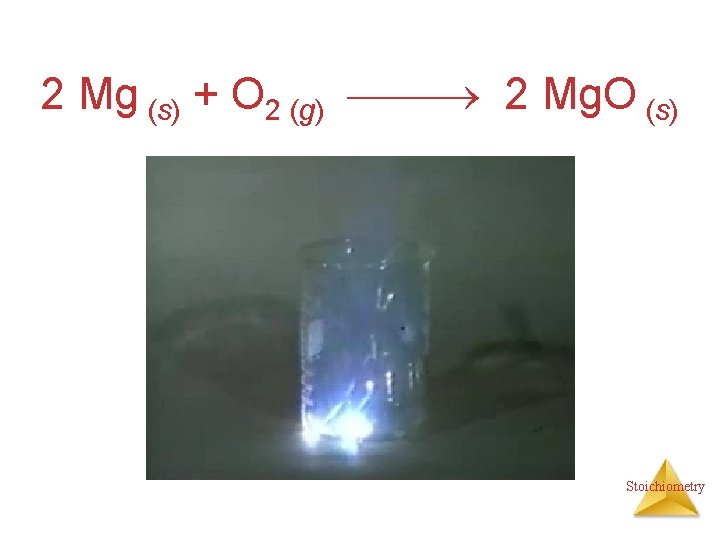2 Mg (s) + O 2 (g) 2 Mg. O (s) Stoichiometry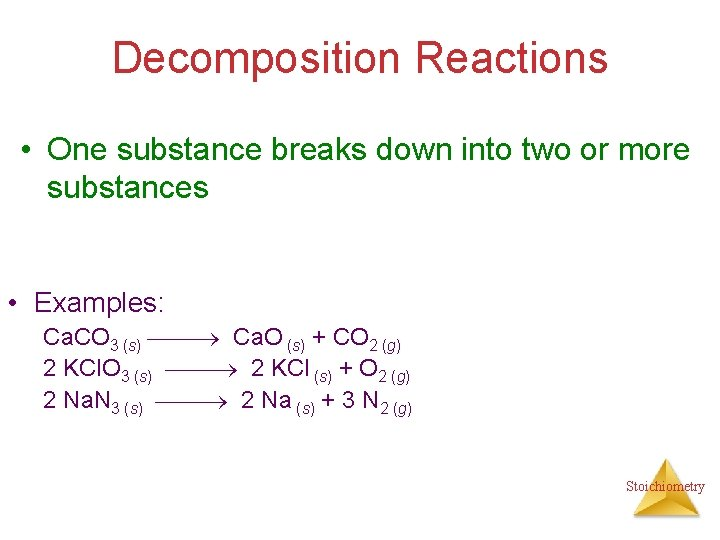Decomposition Reactions • One substance breaks down into two or more substances • Examples: Ca. CO 3 (s) Ca. O (s) + CO 2 (g) 2 KCl. O 3 (s) 2 KCl (s) + O 2 (g) 2 Na. N 3 (s) 2 Na (s) + 3 N 2 (g) StoichiometryCombustion Reactions • Rapid reactions that have oxygen as a reactant sometimes produce a flame • Most often involve hydrocarbons reacting with oxygen in the air to produce CO 2 and H 2 O. • Examples: CH 4 (g) + 2 O 2 (g) CO 2 (g) + 2 H 2 O (g) C 3 H 8 (g) + 5 O 2 (g) 3 CO 2 (g) + 4 H 2 O (g) 2 H 2 + O 2 ------- 2 H 2 O Stoichiometry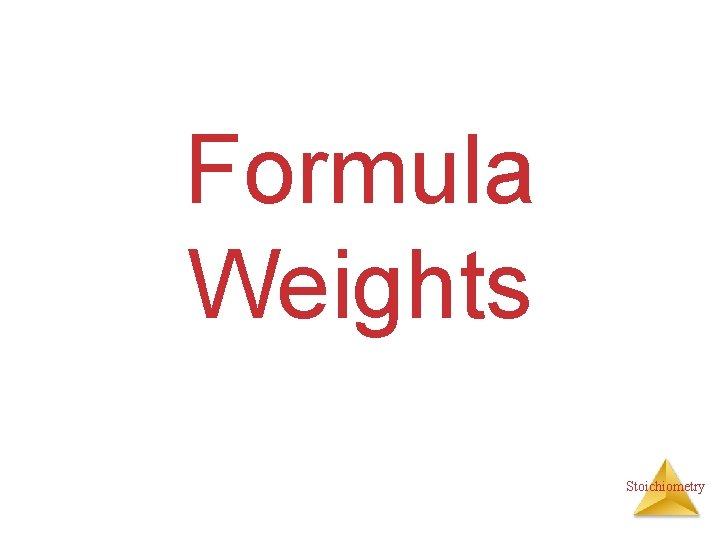Formula Weights StoichiometryThe amu unit • Defined (since 1961) as: • 1/12 mass of the 12 C isotope. • 12 C = 12 amu Stoichiometry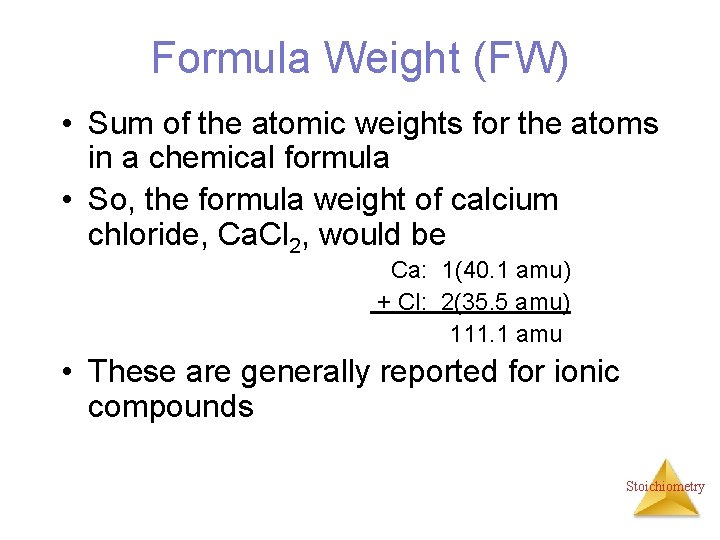Formula Weight (FW) • Sum of the atomic weights for the atoms in a chemical formula • So, the formula weight of calcium chloride, Ca. Cl 2, would be Ca: 1(40. 1 amu) + Cl: 2(35. 5 amu) 111. 1 amu • These are generally reported for ionic compounds StoichiometryMolecular Weight (MW) • Sum of the atomic weights of the atoms in a molecule • For the molecule ethane, C 2 H 6, the molecular weight would be C: 2(12. 0 amu) + H: 6(1. 0 amu) 30. 0 amu StoichiometryPercent Composition One can find the percentage of the mass of a compound that comes from each of the elements in the compound by using this equation: % element = (number of atoms)(atomic weight) (FW of the compound) x 100 StoichiometryPercent Composition So the percentage of carbon and hydrogen in ethane (C 2 H 6, molecular mass = 30. 0) is: %C = %H = (2)(12. 0 amu) (30. 0 amu) (6)(1. 01 amu) (30. 0 amu) = = 24. 0 amu 30. 0 amu 6. 06 amu 30. 0 amu x 100 = 80. 0% x 100 = 20. 0% Stoichiometry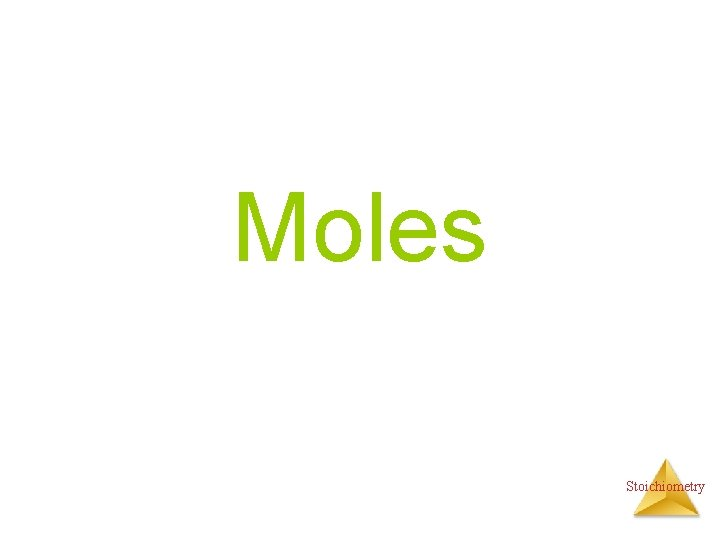Moles StoichiometryAtomic mass unit and the mole • amu definition: 12 C = 12 amu. • The atomic mass unit is defined this way. • 1 amu = 1. 6605 x 10 -24 g • How many 12 C atoms weigh 12 g? • 6. 02 x 1023 12 C weigh 12 g. • Avogadro’s number • The mole Stoichiometry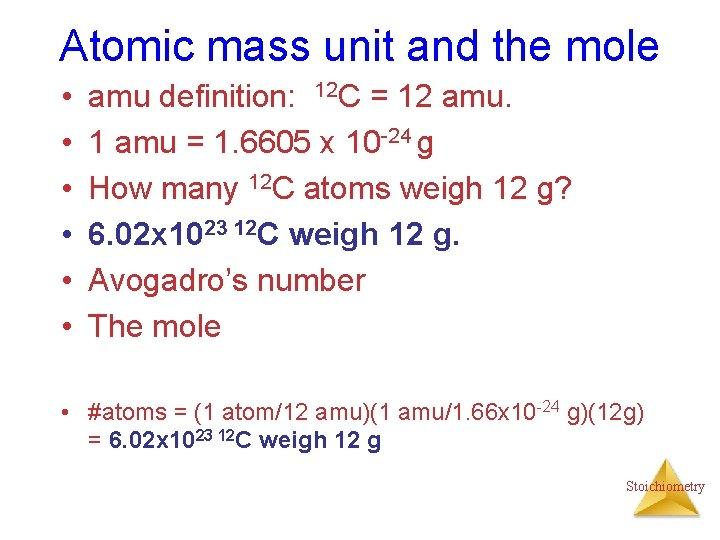Atomic mass unit and the mole • • • amu definition: 12 C = 12 amu. 1 amu = 1. 6605 x 10 -24 g How many 12 C atoms weigh 12 g? 6. 02 x 1023 12 C weigh 12 g. Avogadro’s number The mole • #atoms = (1 atom/12 amu)(1 amu/1. 66 x 10 -24 g)(12 g) = 6. 02 x 1023 12 C weigh 12 g Stoichiometry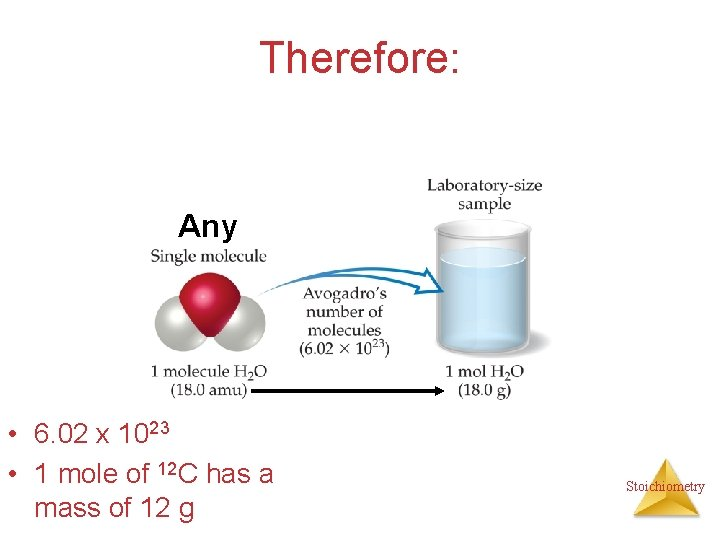Therefore: Any • 6. 02 x 1023 • 1 mole of 12 C has a mass of 12 g Stoichiometry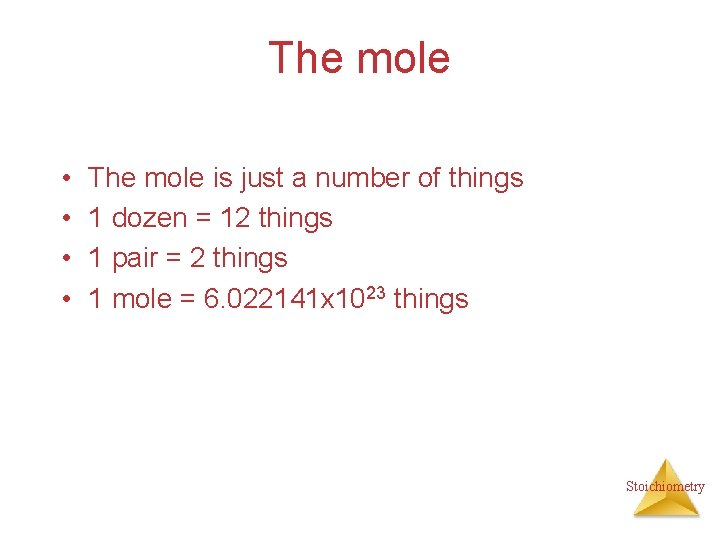The mole • • The mole is just a number of things 1 dozen = 12 things 1 pair = 2 things 1 mole = 6. 022141 x 1023 things StoichiometryMolar Mass The trick: • By definition, this is the mass of 1 mol of a substance (i. e. , g/mol) – The molar mass of an element is the mass number for the element that we find on the periodic table – The formula weight (in amu’s) will be the same number as the molar mass (in g/mol) Stoichiometry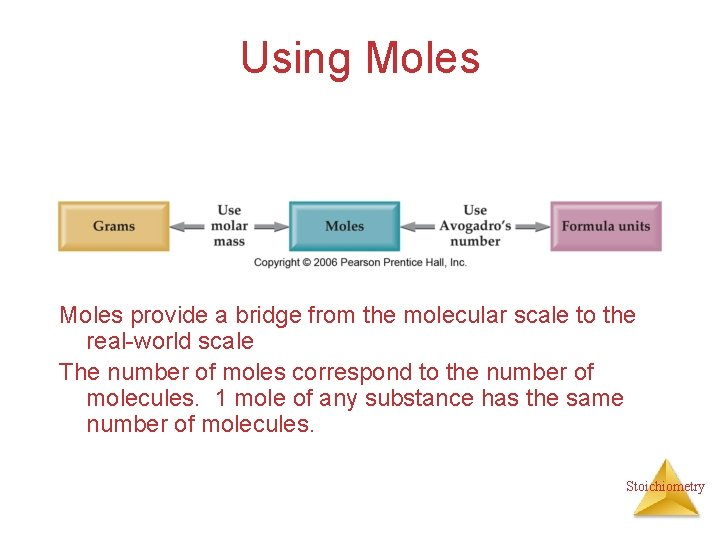Using Moles provide a bridge from the molecular scale to the real-world scale The number of moles correspond to the number of molecules. 1 mole of any substance has the same number of molecules. Stoichiometry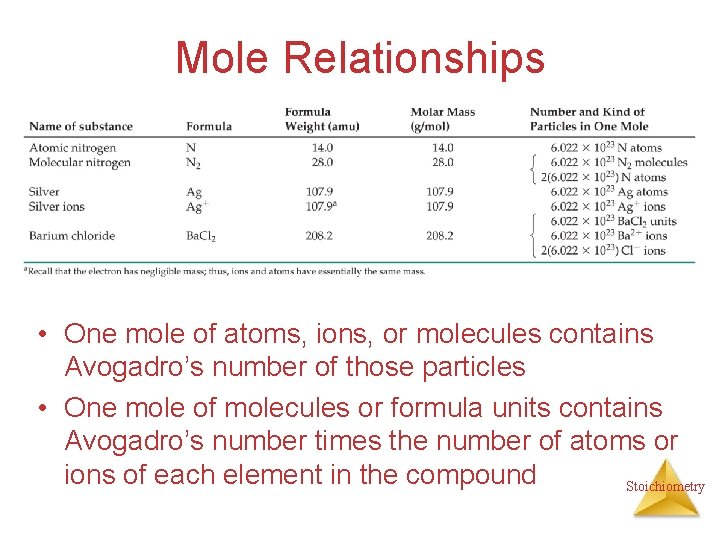Mole Relationships • One mole of atoms, ions, or molecules contains Avogadro’s number of those particles • One mole of molecules or formula units contains Avogadro’s number times the number of atoms or ions of each element in the compound Stoichiometry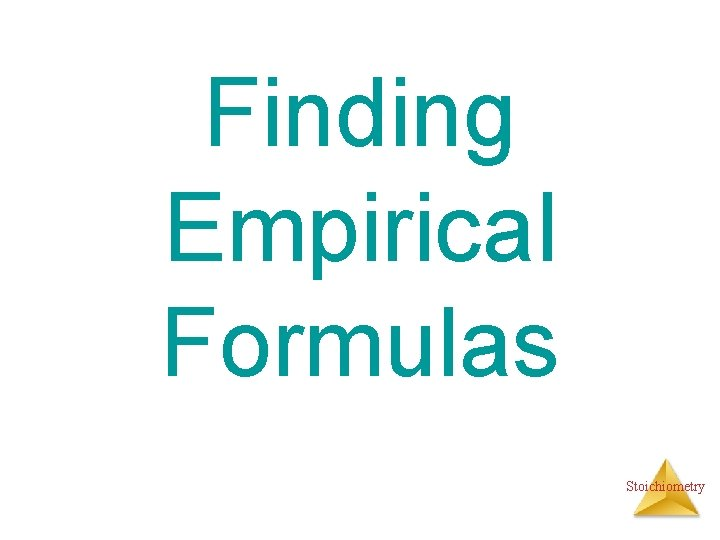Finding Empirical Formulas StoichiometryCombustion Analysis gives % composition C n. H n. O n + O 2 n. CO 2 + 1/2 n. H 2 O • Compounds containing C, H and O are routinely analyzed through combustion in a chamber like this – %C is determined from the mass of CO 2 produced – %H is determined from the mass of H 2 O produced – %O is determined by difference after the C and H have Stoichiometry been determined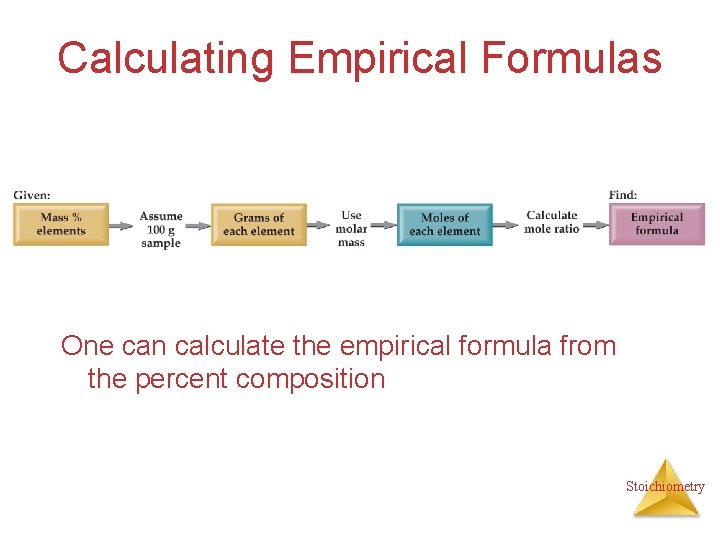Calculating Empirical Formulas One can calculate the empirical formula from the percent composition Stoichiometry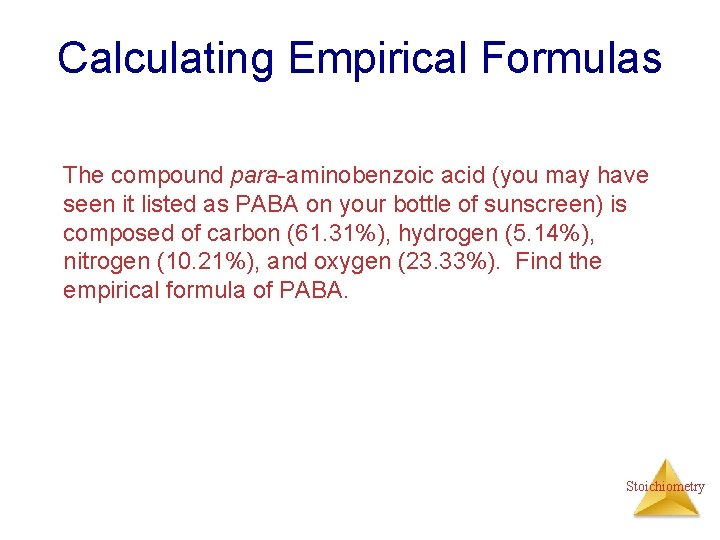Calculating Empirical Formulas The compound para-aminobenzoic acid (you may have seen it listed as PABA on your bottle of sunscreen) is composed of carbon (61. 31%), hydrogen (5. 14%), nitrogen (10. 21%), and oxygen (23. 33%). Find the empirical formula of PABA. Stoichiometry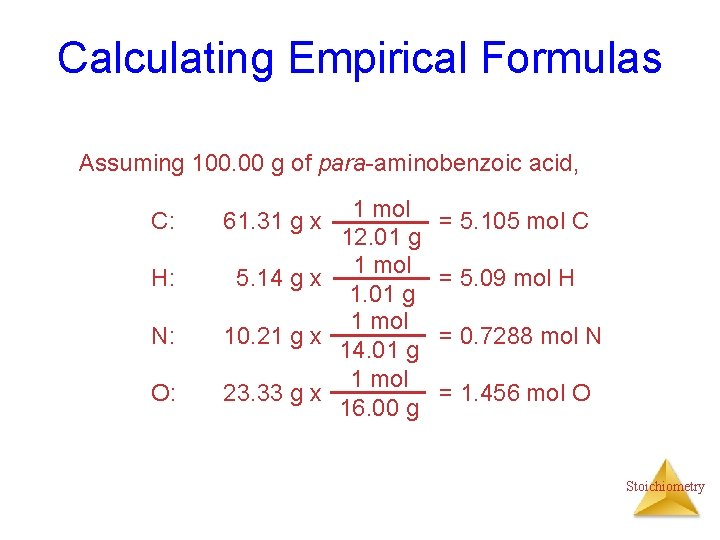Calculating Empirical Formulas Assuming 100. 00 g of para-aminobenzoic acid, C: H: N: O: 1 mol 12. 01 g 1 mol 5. 14 g x 1. 01 g 1 mol 10. 21 g x 14. 01 g 1 mol 23. 33 g x 16. 00 g 61. 31 g x = 5. 105 mol C = 5. 09 mol H = 0. 7288 mol N = 1. 456 mol O Stoichiometry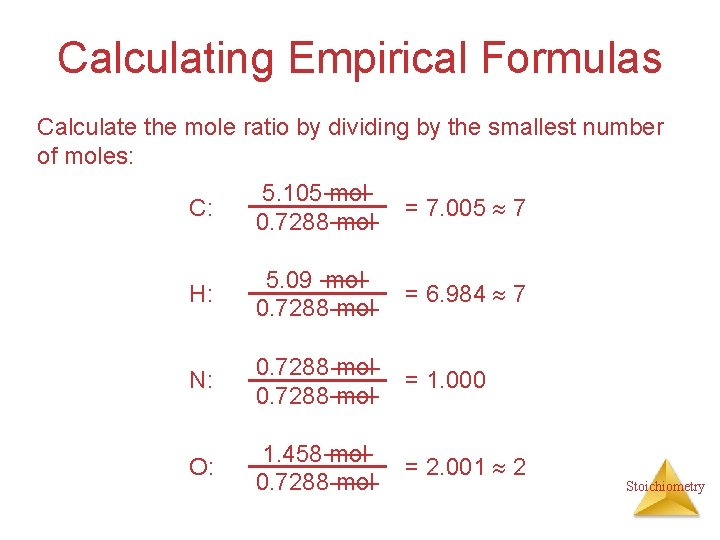Calculating Empirical Formulas Calculate the mole ratio by dividing by the smallest number of moles: C: 5. 105 mol 0. 7288 mol = 7. 005 7 H: 5. 09 mol 0. 7288 mol = 6. 984 7 N: 0. 7288 mol = 1. 000 O: 1. 458 mol 0. 7288 mol = 2. 001 2 Stoichiometry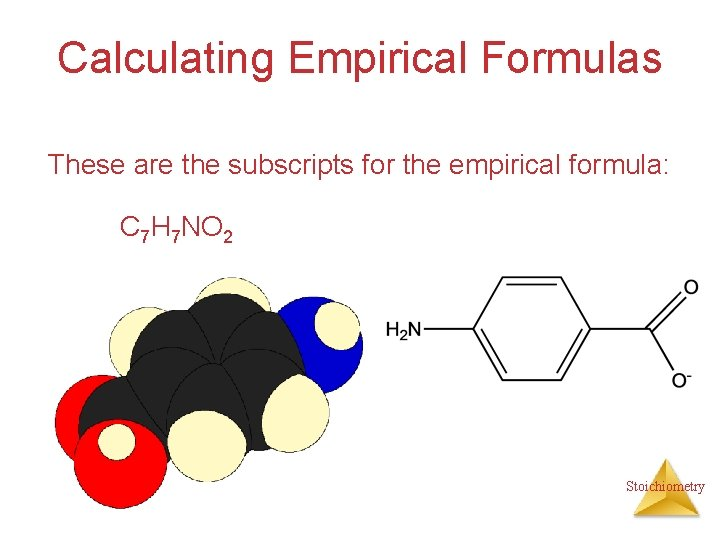Calculating Empirical Formulas These are the subscripts for the empirical formula: C 7 H 7 NO 2 Stoichiometry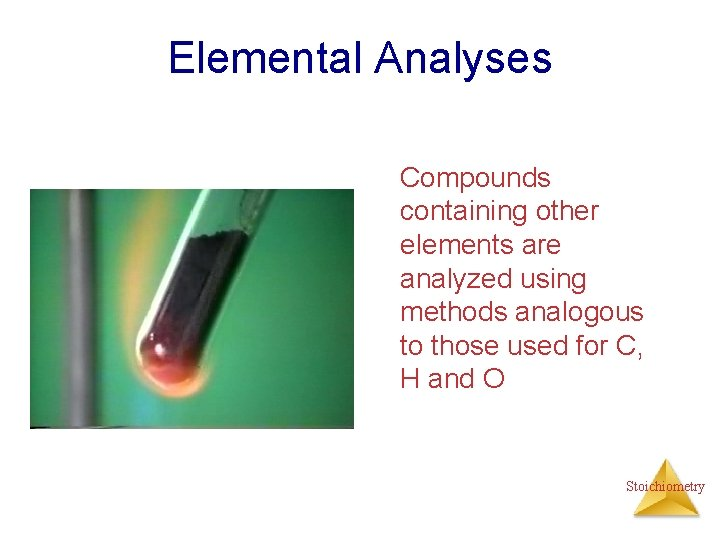Elemental Analyses Compounds containing other elements are analyzed using methods analogous to those used for C, H and O StoichiometryStoichiometric Calculations The coefficients in the balanced equation give the ratio of moles of reactants and products StoichiometryStoichiometric Calculations From the mass of Substance A you can use the ratio of the coefficients of A and B to calculate the mass of Substance B formed (if it’s a product) or used (if it’s a reactant) StoichiometryStoichiometric Calculations Example: 10 grams of glucose (C 6 H 12 O 6) react in a combustion reaction. How many grams of each product are produced? C 6 H 12 O 6(s) + 6 O 2(g) 6 CO 2(g) + 6 H 2 O(l) 10. g ? + ? Starting with 10. g of C 6 H 12 O 6… we calculate the moles of C 6 H 12 O 6… use the coefficients to find the moles of H 2 O & CO 2 and then turn the moles to grams StoichiometryStoichiometric calculations C 6 H 12 O 6 + 10. g MW: 180 g/mol 6 O 2 6 CO 2 ? + + 44 g/mol 6 H 2 O ? 18 g/mol #mol: 10. g(1 mol/180 g) 0. 055 mol #grams: 6(. 055) 6(. 055 mol)44 g/mol 6(. 055 mol)18 g/mol 15 g 5. 9 g Stoichiometry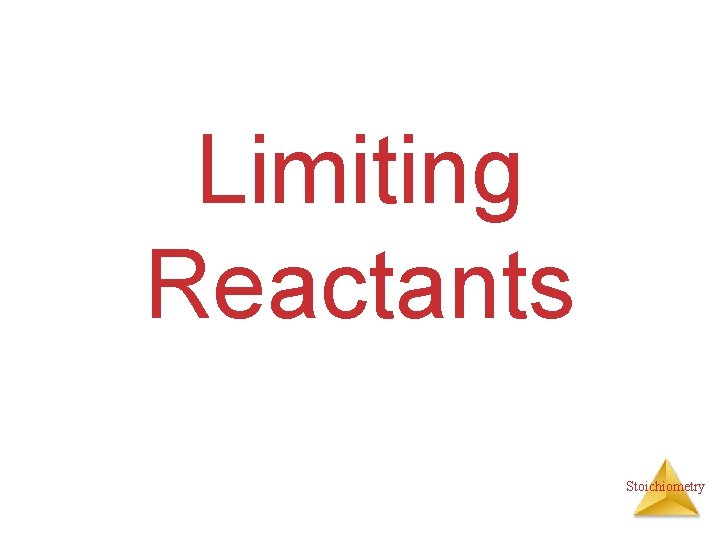Limiting Reactants StoichiometryHow Many Cookies Can I Make? • You can make cookies until you run out of one of the ingredients • Once you run out of sugar, you will stop making cookies StoichiometryHow Many Cookies Can I Make? • In this example the sugar would be the limiting reactant, because it will limit the amount of cookies you can make. StoichiometryLimiting Reactants • The limiting reactant is the reactant present in the smallest stoichiometric amount #moles Left: 2 H 2 14 10 0 + O 2 ----> 7 5 2 2 H 2 O 10 10 Stoichiometry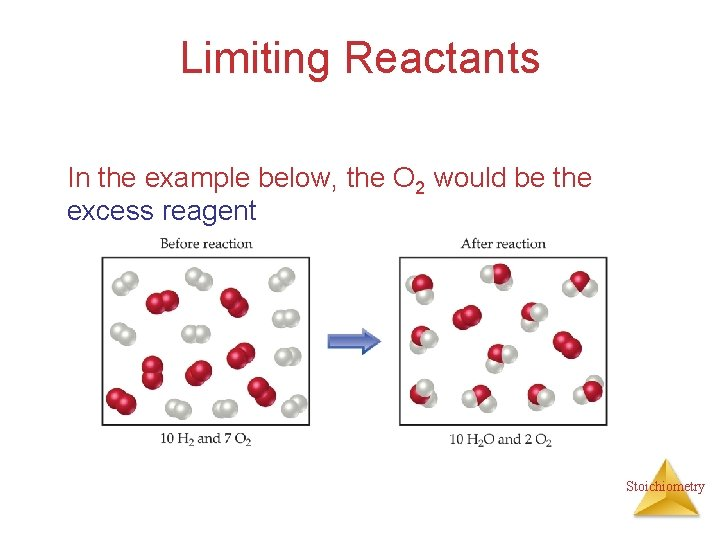Limiting Reactants In the example below, the O 2 would be the excess reagent Stoichiometry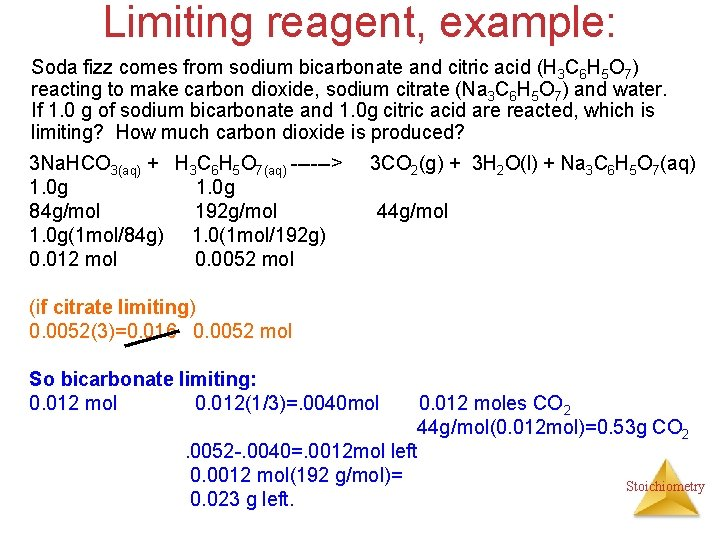Limiting reagent, example: Soda fizz comes from sodium bicarbonate and citric acid (H 3 C 6 H 5 O 7) reacting to make carbon dioxide, sodium citrate (Na 3 C 6 H 5 O 7) and water. If 1. 0 g of sodium bicarbonate and 1. 0 g citric acid are reacted, which is limiting? How much carbon dioxide is produced? 3 Na. HCO 3(aq) + H 3 C 6 H 5 O 7(aq) ------> 1. 0 g 84 g/mol 192 g/mol 1. 0 g(1 mol/84 g) 1. 0(1 mol/192 g) 0. 012 mol 0. 0052 mol 3 CO 2(g) + 3 H 2 O(l) + Na 3 C 6 H 5 O 7(aq) 44 g/mol (if citrate limiting) 0. 0052(3)=0. 016 0. 0052 mol So bicarbonate limiting: 0. 012 mol 0. 012(1/3)=. 0040 mol 0. 012 moles CO 2 44 g/mol(0. 012 mol)=0. 53 g CO 2. 0052 -. 0040=. 0012 mol left 0. 0012 mol(192 g/mol)= Stoichiometry 0. 023 g left.Theoretical Yield • The theoretical yield is the amount of product that can be made – In other words it’s the amount of product possible from stoichiometry. The “perfect reaction. ” • This is different from the actual yield, the amount one actually produces and measures Stoichiometry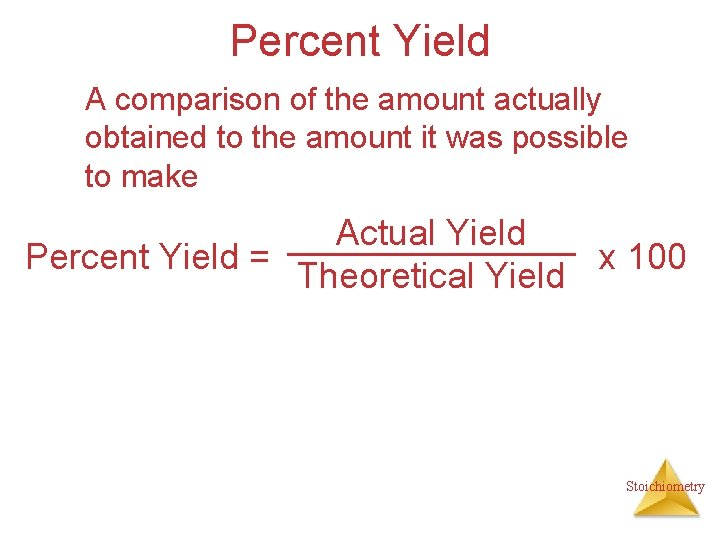Percent Yield A comparison of the amount actually obtained to the amount it was possible to make Actual Yield Percent Yield = x 100 Theoretical Yield StoichiometryExample Benzene (C 6 H 6) reacts with Bromine to produce bromobenzene (C 6 H 6 Br) and hydrobromic acid. If 30. g of benzene reacts with 65 g of bromine and produces 56. 7 g of bromobenzene, what is the percent yield of the reaction? C 6 H 6 + 30. g 78 g/mol 30. g(1 mol/78 g) 0. 38 mol (If Br 2 limiting) 0. 41 mol (If C 6 H 6 limiting) 0. 38 mol Br 2 ------> C 6 H 5 Br + HBr 65 g 56. 7 g 160. g/mol 157 g/mol 65 g(1 mol/160 g) 0. 41 mol 0. 38 mol(157 g/1 mol) = 60. g Stoichiometry 56. 7 g/60. g(100)=94. 5%=95%Example, one more React 1. 5 g of NH 3 with 2. 75 g of O 2. How much NO and H 2 O is produced? What is left? 4 NH 3 + 5 O 2 ----> 1. 5 g 2. 75 g 17 g/mol 32 g/mol 1. 5 g(1 mol/17 g)= 2. 75 g(1 mol/32 g)=. 088 mol. 086 (If NH 3 limiting): . 088 mol. 088(5/4)=. 11 O 2 limiting: . 086(4/5)=. 086 mol. 069 mol(17 g/mol) 1. 2 g 2. 75 g 4 NO ? 30. g/mol + 6 H 2 O ? 18 g/mol . 086 mol(4/5)=. 086(6/5)=. 069 mol. 10 mol. 069 mol(30. g/mol). 10 mol(18 g/mol) 2. 1 g 1. 8 g StoichiometryStoichiometryGun powder reaction • 10 KNO 3(s) + 3 S(s) + 8 C(s) ---- 2 K 2 CO 3(s) + 3 K 2 SO 4(s) + 6 CO 2(g) + 5 N 2(g) • Salt peter sulfur charcoal And heat. • What is interesting about this reaction? What kind of reaction is it? What do you think makes it so powerful? StoichiometryGun powder reaction Oxidizing agent Oxidizing Reducing agent • 10 KNO 3(s) + 3 S(s) + 8 C(s) ---- 2 K 2 CO 3(s) + 3 K 2 SO 4(s) + 6 CO 2(g) + 5 N 2(g) • Salt peter sulfur charcoal And heat. • What is interesting about this reaction? Lots of energy, no oxygen What kind of reaction is it? Oxidation reduction What do you think makes it so powerful and explosive? Makes a lot of gas!!!! StoichiometryWhite phosphorous and Oxygen under water Stoichiometry